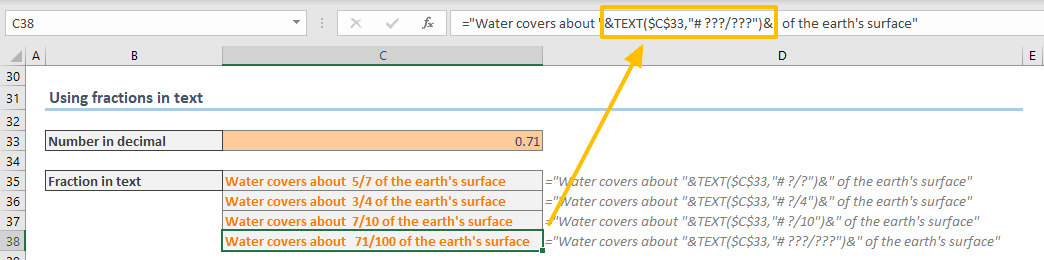Excel usually converts fractions automatically when you enter a date or text value. In this guide, we’re going to show you how to display fractions in Excel.

## Using number formatting to display fractions

Excel stores and calculates any numerical value as decimals. However, you can alter how Excel displays a number. Common examples are displaying currency symbols or deciding the number of decimal digits. The same is true for fractional numbers. You can display any number as a fraction by changing its number formatting.

Follow the steps to apply a fraction number formatting:

1. Select the cells where you want to display fractions.
2. Press Ctrl + 1 or use the right-click menu to open Format Cells.
3. Activate the tab Number.
4. Select Fraction in Category list to see fraction options on the right.
5. Select the option you would like to use.
6. Click OK to apply the number formatting.Here is how Excel displays fractions up to two digits:Excel has 9 predefined fraction formatting options.If these predefined options do not cover your scenarios, you can define your own custom number formatting.

## Custom fractions

You can add custom number formatting options in Excel. Thus, you can set any number as a denominator or create fractions where the top number (numerator) is bigger than the bottom (denominator), e.g., 3/2.

To apply a custom fraction number format, open the Format Cells dialog. This time select Custom in the Category list. You need to enter the custom format code into the Type box.In our example we defined a mixed fraction (fraction with whole number) where the denominator is 256. The dash character (#) represents the whole number, you can use a question mark (?) to define the maximum number of digits of the numerator and the denominator.

Here is a cheat sheet with examples:

 Format Description Example # ?/? A mixed fraction up to single digit denominator. 2/3 1 7/8 # ??/?? A mixed fraction up to double digit denominator. 4/25 2 5/88 ???/??? An improper fraction up to 3 digits. 15/359 254/101 # ??/256 A mixed fraction with fixed denominator 256. 1/256 2 77/256To learn more about number formatting in Excel: Number Formatting in Excel – All You Need to Know

## Using fractions in text

You can set a cell's formatting to show fraction, but the number will lose its formatting when merged with a text. To prevent this, you need to apply formatting with the TEXT function. The TEXT function simply applies the given number formatting to the specified number.

TEXT(number,format)

The format argument accepts the string you can use for custom number formatting.